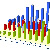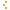MSTE
Office for Mathematics, Science, and Technology Education
College of Education @ University of Illinois

# MSTE Online Resource CatalogData Analysis and Probability

«Show all resources»

«Back to Categories»

Sort By: Title Date

### InteractiveRandom BirthdayBest FitBuffon's NeedleCoin Tossing Game Title Brief Description Category Date Find out just how likely it is that two or more random Birthdays are found on the same day. Data Analysis and Probability 9/9/2016 Generate the best fit of a line. Data Analysis and Probability 6/10/2014 Estimate Pi by calculating the geometrical probability of a needle landing on a line. Data Analysis and Probability 5/28/2014 Learn about probability and hidden states with the MiSTEam Data Analysis and Probability 11/14/2005 Ask Nick to take a random step while he braves the edge of a cliff. Data Analysis and Probability 11/3/2005 A virtual scratch card with user-defined properties. Data Analysis and Probability 4/20/2005 Pick your favorite door and play the game of chance with Monty. Data Analysis and Probability 9/23/2003 Remove your chips with rolls of the dice. Data Analysis and Probability 9/19/2002 Race cars by rolling a die. Data Analysis and Probability 1/28/2002 Plot points on a graph and then fit the data with a polynomial of a chosen degree. Data Analysis and Probability 4/8/2001 Track the spread of a disease among hermits using this interactive Java applet Data Analysis and Probability 6/15/2000 Find the Chi-squared value of drawing a number of random marbles. Data Analysis and Probability 12/8/1999 Launch a rocket if it passes all stages of its flight tests. Data Analysis and Probability 8/8/1999 This page offers statistical programs that can be downloaded directly into the TI-82 or 83. Data Analysis and Probability 2/2/1999 Toss these digital dice and see what numbers roll up. Data Analysis and Probability 6/16/1998 A Game that introduces probability from Hands-on to Resampling Stats Data Analysis and Probability 7/16/1997

### Lessons

Title Brief Description Category Date
Lesson to introduce students both to Mathematica and to the Monte Carlo method of solving probability problems Data Analysis and Probability
10/21/1997
A two day unit plan that incorporates decision-making ideas from statistics into the science classroom. Data Analysis and Probability
10/21/1997
This web page contains nine lessons that use the TI-92 and nine programs for the TI-92. Data Analysis and Probability
7/20/1997
Introduction to Descriptive Statistics with Mode, Median, Mean, and Range Data Analysis and Probability
7/16/1997
This lesson tracks the spread of a disease on a desert island inhabited by hermits. Data Analysis and Probability
5/6/1996
Student activities to explore the concept of expected value Data Analysis and Probability
5/6/1996
Introduction to measure of central tendency and variation. Data Analysis and Probability
4/5/1996
Understand statistical terms such as expectation, significance, and chi-square Data Analysis and Probability
4/5/1996
Your task is to match up the correlation coefficients with the plots. Data Analysis and Probability
2/21/1996
Use the stock market to calculate commissions and costs for buying and selling stock. Data Analysis and Probability
10/2/1995